Perpendicular Bisectors MathBitsNotebook.com Terms of Use   Contact Person: Donna RobertsAlso see "Perpendicular Bisectors in a Triangle" at Segments in Triangles.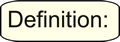A perpendicular bisector of a given line segment is a line (or segment or ray) which is perpendicular to the given segment and intersects the given segment at its midpoint (thus "bisecting" the segment).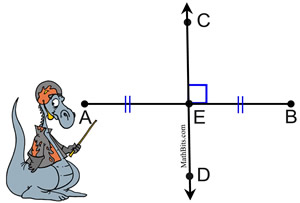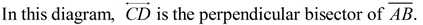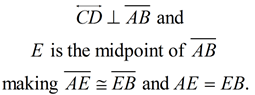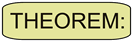The perpendicular bisector of a line segment is the set of all points that are equidistant from its endpoints. To be discussed further in the section on Constructions.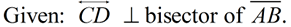Every point on the perpendicular bisector,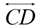, is the same distance from point A as it is from point B. AC = CB AF = FB AG = GB AE = EB AH = HB AJ = JB AD = DB You can think of the points on the perpendicular bisector as being the third vertices of a series of isosceles triangles with vertices A and B.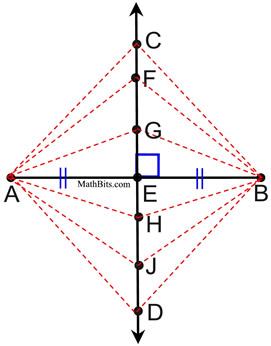Theorem Proof: (transformational method)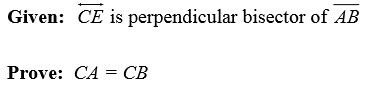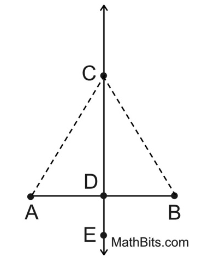1. A perpendicular bisector of a segment (by definition) is a line that is perpendicular to the segment and intersects the segment at its midpoint. 2.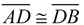because a midpoint of a segment divides the segment into two congruent segments. 3. AD = DB because congruent segments are segments of equal measure. 4. The line of reflection for a segment is the perpendicular bisector of the segment. 5. Under a reflection in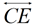, A is mapped onto B, C is mapped onto C, and D is mapped onto D. 6. Under a reflection in,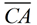is mapped onto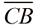. 7. CA = CB since a reflection is a rigid transformation which preserves length.Theorem Proof: (two-column method)Statements Reasons 1.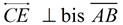1. Given 2.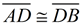2. Segment bisector forms 2 congruent segments. 3. ∠ADC, ∠BDC are right angles 3. Perpendiculars form right angles. 4. ∠ADC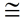∠BDC 4. All right angles are congruent. 5.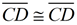5. Reflexive property 6.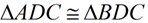6. SAS: If 2 sides and the included angle of one triangle are congruent to the corresponding parts of another triangle, the triangles are congruent. 7.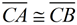7. CPCTC: Corresponding parts of congruent triangles are congruent. 8. CA = CB 8. Congruent segments have equal measure.